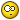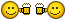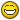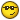# Dynamic block and divide command## Recommended PostsHello all!

I am new to this forum, so please forgive me if I ask stupid questions or simply don't make senseMy work requires me to create programs for our CNC router. It is done in AutoCAD 2008. To help making programs faster I've created series of dynamic blocks. I am attaching a file with one of those blocks.

The file is actually illustrating what I need to do number of times a day.

I would like to make the process even faster and more fool-proof.

The "old way" in file shows what my current way of creating programs.

The "new way" is my attempt to make it faster.

My problem is automatically dividing a line in dynamic block into a variable number of segments that are always equal. Naturally I use divide command after I stretch and explode my dynamic block. It would be much faster if the block would be able to do it all by itself.

My attempt of solving this was to use Visibility Parameter. However, if you look closely at it, you'll notice that if I want to do it for all the possible combinations it will be extremely complicated and easy to mess up.

If only there was a way to have two visibility parameters. Then I'd make one for horizontal holes and another for vertical. Unfortunately I can't.

Does anyone have an idea how to accomplish this? LISP, maybe? Unfortunately, I don't know LISP, although I'd love to learn. I'm a pretty fast learner (I've pretty much taught myself how to make dynamic blocks using only AutoCAD help file). So if you guys know a good guide to start learning LISP, let me know.

Thanks in advance for all the help.

Cheers!Auto hole spacing.dwg

##### Share on other sitesSooo...

few hours with this tutorial and I came up with the following LISP routine.

```(defun C:xh()
(setq entlist(entget(car(entsel))))					;select the horizontal line
(setq point1(cadr(assoc 10 entlist)))					;get x value of start point
(setq point2(cadr(assoc 11 entlist)))					;get x value of end point
(setq xdist(- point2 point1))						;calculate x length
(setq xcount 2)							;defines distance between holes
(setq xdisthole(/ xdist xcount))
(while (< 12.5 xdisthole)
(setq xdisthole(/ xdist xcount))
(setq xcount(+ xcount 1))
)
(princ "\n Line length is ")(princ xdist)(princ ".")			;outputs the information
(princ "\n Divide line into ")(princ (- xcount 1))(princ " pieces.")
(princ "\n Distance between holes is ")(princ xdisthole)(princ ".")
(setq xcount 2)							;resets the xcount to 2 so I can use this program again without reloading
(princ)
)```

It let's me choose a horizontal line and divides it into equal parts that meet my criteria.

What I need now is to figure out how to make it copy a circle along the line every "xdisthole" distance.

Can anyone point me in the right direction?

Thanks!

##### Share on other sitesWell...

This is what I came up with. (If anyone is even interested in it anyway...)

Few more hours of reading and experimenting and here we are! I achieved exactly what I was aiming for.I realize that my code is probably very "unprofessional" but, hey, it's my first program ever. At least it's not another "Hello, world"...Good luck to all us, beginners!```(defun C:dg()
(setq entlist(entget(car(entsel))));select the line
(setq point1x(cadr(assoc 10 entlist)));get x value of start point
(setq point1x_str(rtos point1x 2 6));converts value to string
(setq point1x(atof point1x_str));converts string back to real number
(setq point1y(caddr(assoc 10 entlist)));get y value of start point
(setq point1y_str(rtos point1y 2 6))
(setq point1y(atof point1y_str))
(setq point2x(cadr(assoc 11 entlist)));get x value of end point
(setq point2x_str(rtos point2x 2 6))
(setq point2x(atof point2x_str))
(setq point2y(caddr(assoc 11 entlist)));get y value of end point
(setq point2y_str(rtos point2y 2 6))
(setq point2y(atof point2y_str))
(if (< point1x point2x);checks the direction of a line and then calculates X length
(setq xdist(- point2x point1x))
(setq xdist(- point1x point2x)))
(if (< point1y point2y);checks the direction of a line and then calculates Y length
(setq ydist(- point2y point1y))
(setq ydist(- point1y point2y)))
(setq xcount 2);defines initial x distance between holes
(setq ycount 2);defines initial y distance between holes
(cond;figures out if the line is horizontal, or vertical, or none
( (= ydist 0);horizontal
(setq xdisthole(/ xdist xcount));calculates max distance between holes, but less than 12.5
(while (< 12.5 xdisthole)
(setq xdisthole(/ xdist xcount))
(setq xcount(+ xcount 1)))
(princ "\n Line length is ")(princ xdist)(princ ". ");outputs the information
(princ "Divide line into ")(princ (- xcount 1))(princ " pieces. ")
(princ "Distance between holes is ")(princ xdisthole)(princ ".")
(princ "\n")
(if (< point1x point2x)
(progn
(while (<= 1 xcount);draws holes in positive direction
(setq xhole(list point1x point1y))
(command "circle" xhole "D" "0.344")
(setq point1x(+ point1x xdisthole))
(setq xcount(- xcount 1))
))
(progn
(while (<= 1 xcount);draws holes in negative direction
(setq xhole(list point1x point1y))
(command "circle" xhole "D" "0.344")
(setq point1x(- point1x xdisthole))
(setq xcount(- xcount 1))
))
)
(setq xcount 2);reset
)
( (= xdist 0)
(setq ydisthole(/ ydist ycount))
(while (< 10.5 ydisthole)
(setq ydisthole(/ ydist ycount))
(setq ycount(+ ycount 1)))
(princ "\n Line length is ")(princ ydist)(princ ". ");outputs the information
(princ "Divide line into ")(princ (- ycount 1))(princ " pieces. ")
(princ "Distance between holes is ")(princ ydisthole)(princ ".")
(princ "\n")
(if (< point1y point2y)
(progn
(while (<= 1 ycount);draws holes in positive direction
(setq yhole(list point1x point1y))
(command "circle" yhole "D" "0.344")
(setq point1y(+ point1y ydisthole))
(setq ycount(- ycount 1))
))
(progn
(while (<= 1 ycount);draws holes in negative direction
(setq yhole(list point1x point1y))
(command "circle" yhole "D" "0.344")
(setq point1y(- point1y ydisthole))
(setq ycount(- ycount 1))
))
)
(setq ycount 2)
)
( T
(princ "Invalid line. Check if it's vertical or horizontal!")
)
)
(princ)
)
```

## Join the conversation

You can post now and register later. If you have an account, sign in now to post with your account.
Note: Your post will require moderator approval before it will be visible.×   Pasted as rich text.   Restore formatting

Only 75 emoji are allowed.